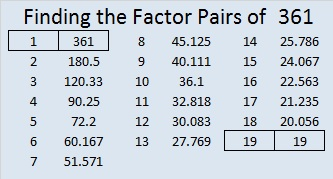# Mysterious 1023

2⁹ + 2⁸ + 2⁷ + 2⁶ + 2⁵ + 2⁴ + 2³ + 2² + 2¹ + 2⁰ = 1023. That makes 1023 a pretty cool and rather mysterious number.

This puzzle that I’ve numbered 1023 is pretty cool and mysterious, too. I’m sure you will enjoy solving it if you only use logic to find the solution.Print the puzzles or type the solution in this excel file: 10-factors-1019-1027

Here are some other fascinating facts about the number 1023:

It is formed by using a zero and three other consecutive numbers, so it is divisible by 3.

1 – 0 + 2 – 3 = 0, so 1023 is divisible by eleven.

31 × 33 = 1023 so (32 – 1)(32 + 1) = 1023, AND it is 32² – 1, making it one away from the next square number!

It is the sum of five consecutive prime numbers:
193 + 197 + 199 + 211 + 223 = 1023

1023 looks quite interesting when it is written in several different bases:
First of all, it’s 1111111111 in BASE 2 because it is the sum of the all those powers of 2 from 0 to 9 that were included at the top of this post.

It’s also 33333 in BASE 4 because 3(4⁴ + 4³ + 4² + 4¹ + 4⁰) = 3(341) = 1023.
That also means that 3(2⁸ + 2⁶ + 2⁴ + 2² + 2⁰) = 1023

It’s 393 in BASE 17 because 3(17²) + 9(17) + 3(1) = 1023,
VV in BASE 32 (V is 31 base 10) because 31(32) + 31(1) = 31(33) = 1023, and
V0 in BASE 33 because 31(33) = 1023

• 1023 is a composite number.
• Prime factorization: 1023 = 3 × 11 × 31
• The exponents in the prime factorization are 1, 1, and 1. Adding one to each and multiplying we get (1 + 1)(1 + 1)(1 + 1) = 2 × 2 × 2 = 8. Therefore 1023 has exactly 8 factors.
• Factors of 1023: 1, 3, 11, 31, 33, 93, 341, 1023
• Factor pairs: 1023 = 1 × 1023, 3 × 341, 11 × 93, or 31 × 33
• 1023 has no square factors that allow its square root to be simplified. √1023 ≈ 31.98437# 675 and Level 2

675 is made from 3 consecutive numbers so 675 is divisible by 3. Since the middle number of those consecutive numbers, 6, is divisible by 3, we know that 675 is also divisible by 9.

Since the last two digits of 675 is a multiple of 25, we know that 675 is divisible by 25.

Since 5 and 25 are two of its factors, 675 is the hypotenuse of two Pythagorean triples: 405-540-675 and 189-648-675. What is the greatest common factor of each set of three numbers?

The numbers in one of 675’s factor pairs, 25 and 27, are each exactly one number away from 26, their average. That means we are just one number away from 26².

Thus, 675 equals 26² – 1² which can be factored into (26 + 1)(26 – 1) so (26 + 1)(26 – 1) = 27 x 25 = 675.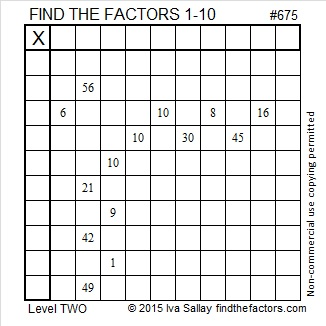Print the puzzles or type the solution on this excel file: 10 Factors 2015-11-09

—————————————————————————————————

• 675 is a composite number.
• Prime factorization: 675 = 3 x 3 x 3 x 5 x 5, which can be written 675 = (3^3) x (5^2)
• The exponents in the prime factorization are 3 and 2. Adding one to each and multiplying we get (3 + 1)(2 + 1) = 4 x 3 = 12. Therefore 675 has exactly 12 factors.
• Factors of 675: 1, 3, 5, 9, 15, 25, 27, 45, 75, 135, 225, 675
• Factor pairs: 675 = 1 x 675, 3 x 225, 5 x 135, 9 x 75, 15 x 45, or 25 x 27
• Taking the factor pair with the largest square number factor, we get √675 = (√225)(√3) = 15√3 ≈ 25.98076.—————————————————————————————————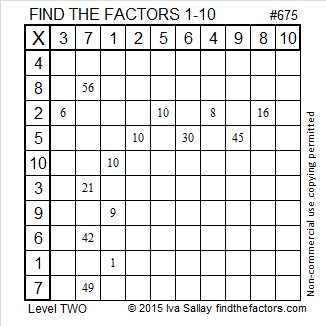# 575 and Level 4

575 is the hypotenuse of two Pythagorean triples: 345-460-575 and 161-552-575. What is the greatest common factor of each of those triples?

575 = 23 x 25, which can be written (24 – 1)(24 + 1) or (24^2) – 1.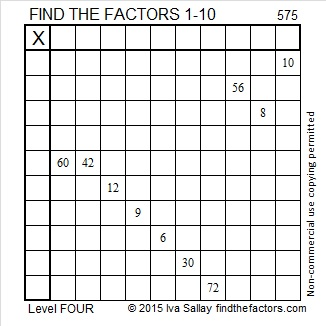Print the puzzles or type the solution on this excel file: 10 Factors 2015-08-03

—————————————————————————————————

• 575 is a composite number.
• Prime factorization: 575 = 5 x 5 x 23, which can be written 575 = (5^2) x 23
• The exponents in the prime factorization are 2 and 1. Adding one to each and multiplying we get (2 + 1)(1 + 1) = 3 x 2  = 6. Therefore 575 has exactly 6 factors.
• Factors of 575: 1, 5, 23, 25, 115, 575
• Factor pairs: 575 = 1 x 575, 5 x 115, or 23 x 25
• Taking the factor pair with the largest square number factor, we get √575 = (√25)(√23) = 3√23 ≈ 23.9791576—————————————————————————————————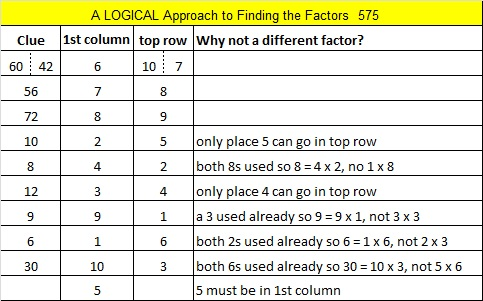# 361 Do You See a Pattern?

When 2^361 is divided by 361, the remainder is 116, not 2. That means that 361 is definitely a composite number. Its factors are listed at the end of this post.

361 isn’t used as often, but it is just as special as some of the numbers in the table below:The pattern can also be seen along the diagonals in this ordinary multiplication table:This pattern could be very helpful to students who are learning to multiply. I have seen plenty of students who knew 7 x 7 = 49, but couldn’t remember what 6 x 8 is.

Years after I learned the multiplication facts, I learned how to multiply binomials in an algebra class. I learned about the difference of two squares. In the example below one of the squares is n² and the other square is 1² which is equal to 1. I learned that the equation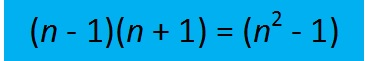is true for ALL numbers, but nobody pointed out any practical examples to make it more meaningful. The table at the top of the page contains twelve practical examples. Let’s see how you do applying it to products of a few larger numbers.

Sometimes we find easy ways to remember certain products like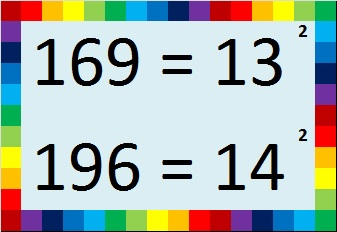We can use those products to help us remember other products easily by applying the difference of two squares. Try these: (Yes, you can easily do them without a calculator!)

• 13 x 13 = 169. How much is 12 x 14?
• 14 x 14 = 196. How much is 13 x 15?
• 20 x 20 = 400. How much is 19 x 21?
• If you know that the first ten powers of 2 are 2, 4, 8, 16, 32, 64, 128, 256, 512, 1024, then it’s easy to remember that 16 x 16 = 256. How much is 15 x 17?
• What if we go in the opposite direction…It isn’t too hard to multiply 18 x 20 in your head to get 360. How much is 19 x 19?
• 22 x 20 = 440 was also easy to find. How much is 21 x 21?
• 30 x 30 = 900. How much is 29 x 31?
• 100 x 100 = 10,000. How much is 99 x 101?Did you figure out what 361 has to do with this pattern? It is a perfect square just like 1, 4, 9, 16, and 25. Here is its factoring information:

• 361 is a composite number.
• Prime factorization: 361 = 19^2
• The exponent in the prime factorization is 2. Adding one we get (2 + 1) = 3. Therefore 361 has exactly 3 factors.
• Factors of 361: 1, 19, 361
• Factor pairs: 361 = 1 x 361 or 19 x 19
• 361 is a perfect square. √361 = 19